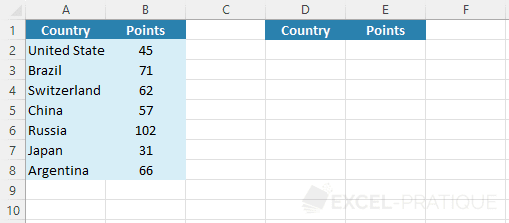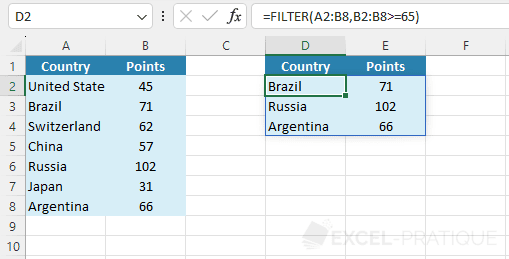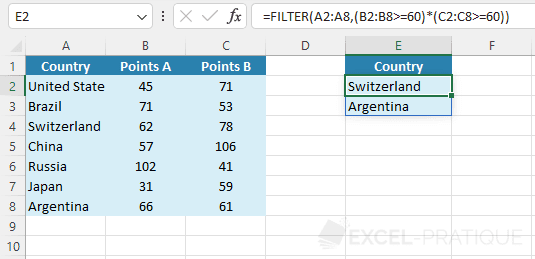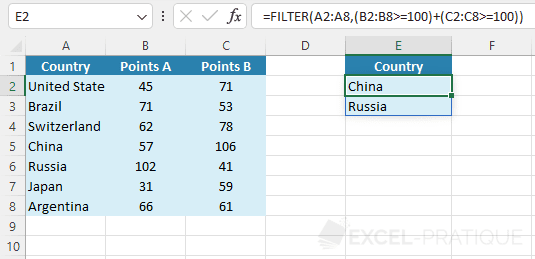# Excel Function: FILTER

The Excel function FILTER allows you to filter a range of cells based on one or more criteria.

Usage:

`=FILTER(array, criteria)`

or

`=FILTER(array, criteria, if_no_result)`

## Usage example

In this example, the goal is to filter the data to get the list of countries with a score of 65 or higher:Select the FILTER function and enter the cell range A2:B8 (to return the countries + points) and the criteria B2:B8>=65 (to test the points):

``=FILTER(A2:B8,B2:B8>=65)``To avoid getting an error if there is no result, fill in the third argument (with for example the value "").

If necessary, you can use the SORT function to sort the table returned by the FILTER function. For example, a sort by descending points:

``=SORT(FILTER(A2:B8,B2:B8>=65),2,-1)``## Filter based on several criteria

To get the list of countries that scored 60 points or more in the 2 columns (so B2:B8>=60 and C2:C8>=60), add these 2 criteria in parentheses and multiply them:

``=FILTER(A2:A8,(B2:B8>=60)*(C2:C8>=60))``## Filter based on at least one criteria

To get the list of countries that scored 100 points or more in at least one of the 2 columns (so B2:B8>=100 or C2:C8>=100), add these 2 criteria in parentheses and add them together:

``=FILTER(A2:A8,(B2:B8>=100)+(C2:C8>=100))``